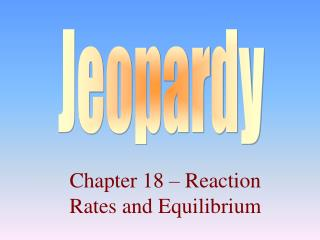DownloadDownload PresentationChapter 18 – Reaction Rates and Equilibrium

# Chapter 18 – Reaction Rates and Equilibrium

Télécharger la présentation## Chapter 18 – Reaction Rates and Equilibrium

- - - - - - - - - - - - - - - - - - - - - - - - - - - E N D - - - - - - - - - - - - - - - - - - - - - - - - - - -
##### Presentation Transcript

1. Jeopardy Chapter 18 – Reaction Rates and Equilibrium

2. Rates of Reactions Equilibrium Entropy and Free Energy Le Chatelier’s Principle 100 100 100 100 200 200 200 200 300 300 300 300 400 400 400 400 500 500 500 500

3. Row 1, Col 1 Temperature or concentration of reactants The rate of a chemical reaction may be increased by increasing the _______ and / or ________.

4. 1,2 They are equal. When a reaction reaches equilibrium, what can you say about the rate of the forward reaction and reverse reaction?

5. 1,3 Entropy (S) What is the name and the symbol of the term used to describe the ‘disorder’ of a process?

6. 1,4 Left Shift Decreasing the temperature of the following reaction at equilibrium will cause it to shift in which direction? N2(g) + 3H2(g) + Energy  2NH3(g)

7. 2,1 Increase As the particle size of a reactant decreases, the rate of reaction will __________.

8. 2,2 Reactants are favored at equilibrium. What can you conclude about a chemical reaction that has reached equilibrium were the equilibrium constant (Keq) is less than one?

9. 2,3 Increase Is there an increase or decrease in The entropy in the following process? CaCO3(s)  CaO(s) + CO2(g)

10. 2,4 It will shift to the left. What will happen to the position of the equilibrium when the the pressure is raised on the following chemical reaction? PCl5(g)  PCl3(g) + Cl2(g)

11. 3,1 It lowers the activation energy. How does a catalyst speed up a chemical reaction?

12. 3,2 Keq = [CH4] [H2S]2 [H2]4 [CS2] Write the equilibrium expression for the following chemical reaction; 4H2(g) + CS2(g)  CH4(g) + 2H2S(g)

13. 3,3 Entropy (S) and Enthalpy (H) Which two factors together determine if a reaction is spontaneous or not?

14. 3,4 No effect, Keq = [O2]2 What effect will the addition of KClO4 have on the following Equilibrium? KClO4(s)  KCl(s) + 2O2(g)

15. 4,1 Molarity / time What is the unit usually used to express the rate of a chemical reaction?

16. 4,2 Ksp = [Pb+4] [CO3-2]2 Write the solubility product expression, Ksp, for the dissolution of lead (IV) carbonate.

17. 4,3 It may or may not be spontaneous. If the entropy and enthalpy of a chemical reaction both decrease, what can you conclude about the spontaneity of the reaction?

18. 4,4 left Reducing the temperature on the following reaction will cause it to shift in which direction? N2(g) + 3H2(g) + Energy  2NH3(g)

19. 5,1 Rate = k [N2] [H2]3 Write the rate law for the following chemical reaction; N2(g) + 3H2(g)  2NH3(g)

20. 5,2 The addition of Cl- would cause PbCl2 to precipitate. What will the addition of NaCl do To a saturated solution of PbCl2?

21. 5,3 will The following chemical reaction (will / will not) be spontaneous. C3H8(g) + 5O2(g)  3CO2(g) + 4H2O(g) + Energy

22. 5,4 No effect What effect would adding water to the following reaction have on its equilibrium position? Na(s) + 2H2O(l)  2NaOH(aq) + H2(g)

23. Final Jeopardy • What is the concentration of carbonate ions in a saturated solution of SrCO3? Ksp = 9.3 x 10-10